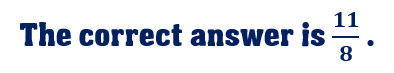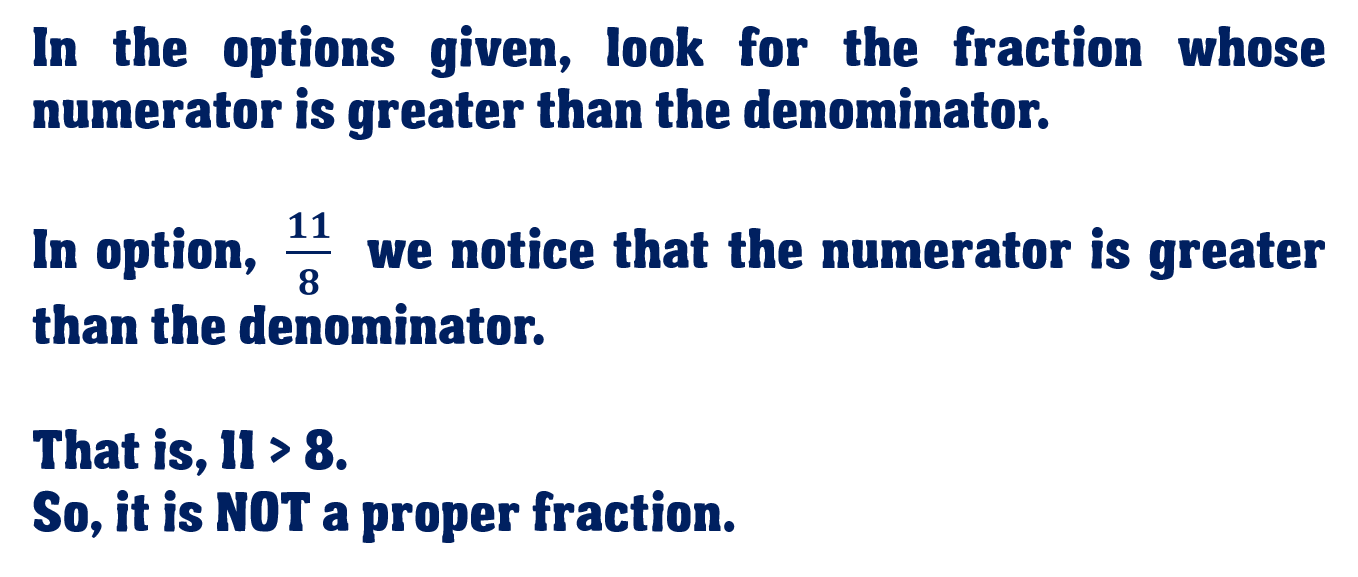1
visibility

Which one of the following is NOT a proper fraction?

• \$latex \dfrac{3}{5}\$

• \$latex \dfrac{4}{9}\$

• \$latex \dfrac{15}{17}\$

• \$latex dfrac{11}{8}\$Please Note:

A proper fraction is a fraction whose numerator (the top number) is less than the denominator (the bottom number).

An improper fraction is a fraction whose numerator (the top number) is greater than the denominator (the bottom number).

Solution: